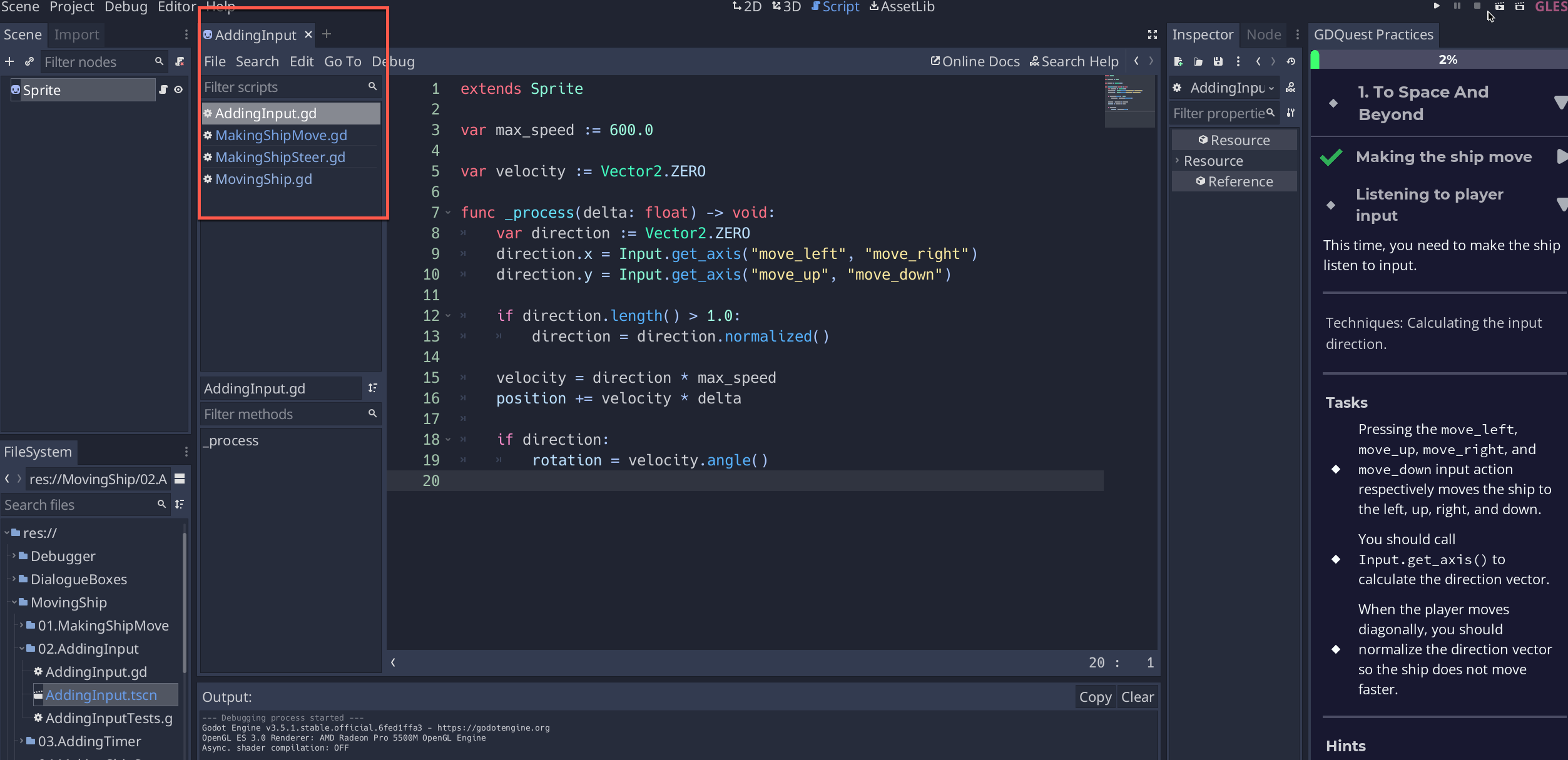T
TiSiLud

# Should I be able to run this right away?

I'm unsure if I should be able to run this now, I have coded along with you, I have the the MakingShipMove.gd script along with:

• MakingShipSteer.gd
• MovingShip.gd

In this video you only have MakingShipMove.gd - is that all I should have?

My code is exactly the same as yours (I have used underscores to illustrate indentations - these are not present in my actual script):

extends Sprite

var max_speed := 600.0

var velocity := Vector2.ZERO

func _process(delta: float) -> void:

____var direction := Vector2.ZERO

____direction.x = Input.get_axis("move_left", "move_right")

____direction.y = Input.get_axis("move_up", "move_down")

____if direction.length() > 1:

________direction = direction.normalized()

____velocity = direction * max_speed

____position += velocity * delta

____if direction:

________rotation = velocity.angle()

The only test which is passing is "Get axis calls Use Correct Move Actions, the rest fail.:

1. We didn't find a line of code that normalized the direction of the vector. Did you assign direction.normalized()?

2. You need to use the Input.get_axis() function to get the ships movement direction.

3. One or more more of the four direction keys did not move the ship.

4. The velocity should be equal to direction * max_speed. It seems that is not the case, did you perhaps multiply the max_speed by delta?

I have deleted and re-written the code but I cannot get these tests to pass - should I have the other scripts in there already - its the only thing I can see that would be causing this?

Thanks :)

•Xananax replied

Hey! I'm not precisely sure I understand the issue; I'm going to make some assumptions:

1. Assumption 1: You're passing the exercises
2. Assumption 2: You're at exercise 1.2: "listening to player inputs"
3. Assumption 3: You wrote the code you're referencing in a new file you created called MovingShip.gd

If all my assumptions are correct, you simply aren't using the correct file! The instructions might be unclear, sorry for that. You need to write the code in the file provided in the exercise!

When you click "Listening to Player Input" in the right panel, it should automatically open the correct scene and the correct script. Is this happening?

If yes, you should see the following:

1 love
• T
TiSiLud replied

Ah sorry I don't think I am being clear.

Assumption 1 and 2 are correct, however 3 is not - I didn't create the file. I am just using what is already loaded when I click "Listening to Player Input" in the right panel - I've added to the script (along with you), but the tests aren't passing. I thought it may be because I have these other scripts (on the left, below AddingInput.gd:I checked the code over several times, it looks exactly like yours however when I run the program I get the following failures:

OK, I just ran the program but reduced the window size from full screen so that I could use Snagit to capture an image of the test results, and it passed.

Isn't MacOS wonderful XD

Thanks for the help and sorry for wasting your time, the course has been great so far!

•Xananax replied

That isn't a waste of time, if that happens to you it means we need to look out for it! There must be something in the tests that assume a specific window or screen size. We'll need to check. Thank you for the report!

• M
Mysterion0 replied

I'm running into something similar under Linux.  I attempted to do the "Listening to player input" practice and all the checks fail but "Direction Uses Get Axis Function" and "Get Axis Calls Use Correct Move Actions".    I have the code below.  The ship looks like its looping through the multiple directions before the checks fail.

extends Sprite

var max_speed := 600.0

var velocity := Vector2.ZERO

func _process(delta: float) -> void:

# Calculate the input direction using Input.get_axis().

var direction := Vector2.ZERO

direction.x = Input.get_axis("move_left","move_right")

direction.y = Input.get_axis("move_up","move_down")

# Limit the direction vector's length by normalizing the vector.

# You need to replace "pass" below.

if direction.length() > 1.0:

direction.normalized()

# Complete this line to move the ship.

velocity *= direction * max_speed

position += velocity * delta

if direction:

rotation = velocity.angle()

1 love
•Xananax replied

That code seems completely fine to me. Can you try resizing the window like the previous user? It seems like we have a bug in our code in how we verify things, and we aren't taking into account certain resolutions.

1 love
• M
Mysterion0 replied

Hi Xananax,

I believe I found the errors.  I checked the unit testing script that came with the practice.

I  had direction.normalized() where it should of assigned the return of the method so:

direction.normalized()     ---To-->    direction = direction.normalized()

This makes sense since normalized()  only returns and doesn't change direction in its method.

The other change:

velocity *= direction * max_speed    ---To-->    velocity = direction * max_speed

And that makes sense since I was multiplying a zero vector (0,0) (velocity = Vector2.ZERO) to velocity which canceled out velocity.  I think that was a typo on my part.

•